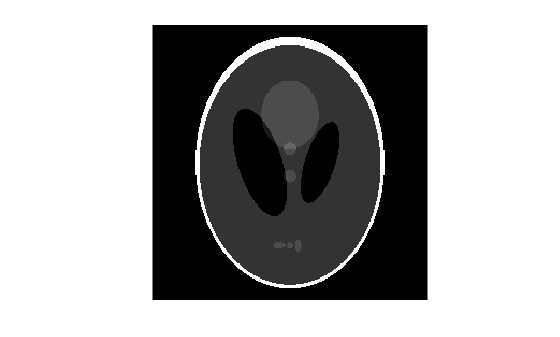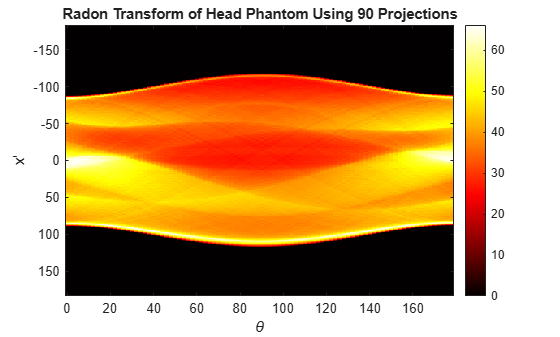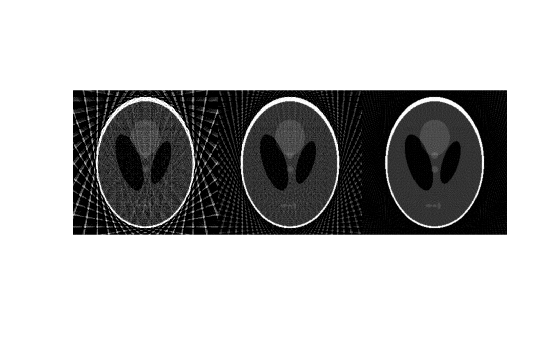The iradon function inverts the Radon transform and can therefore be used to reconstruct images.

As described in Radon Transform, given an image I and a set of angles theta, the radon function can be used to calculate the Radon transform.

R = radon(I,theta);

The function iradon can then be called to reconstruct the image I from projection data.

IR = iradon(R,theta);

In the example above, projections are calculated from the original image I.

Note, however, that in most application areas, there is no original image from which projections are formed. For example, the inverse Radon transform is commonly used in tomography applications. In X-ray absorption tomography, projections are formed by measuring the attenuation of radiation that passes through a physical specimen at different angles. The original image can be thought of as a cross section through the specimen, in which intensity values represent the density of the specimen. Projections are collected using special purpose hardware, and then an internal image of the specimen is reconstructed by iradon. This allows for noninvasive imaging of the inside of a living body or another opaque object.

iradon reconstructs an image from parallel-beam projections. In parallel-beam geometry, each projection is formed by combining a set of line integrals through an image at a specific angle.

The following figure illustrates how parallel-beam geometry is applied in X-ray absorption tomography. Note that there is an equal number of n emitters and n sensors. Each sensor measures the radiation emitted from its corresponding emitter, and the attenuation in the radiation gives a measure of the integrated density, or mass, of the object. This corresponds to the line integral that is calculated in the Radon transform.

The parallel-beam geometry used in the figure is the same as the geometry that was described in Radon Transform. f(x,y) denotes the brightness of the image and ${R}_{\theta }\left(x\prime \right)$ is the projection at angle theta.

Parallel-Beam Projections Through an ObjectAnother geometry that is commonly used is fan-beam geometry, in which there is one source and n sensors. For more information, see Fan-Beam Projection. To convert parallel-beam projection data into fan-beam projection data, use the para2fan function.

#### Improving the Results

iradon uses the filtered back projection algorithm to compute the inverse Radon transform. This algorithm forms an approximation of the image I based on the projections in the columns of R. A more accurate result can be obtained by using more projections in the reconstruction. As the number of projections (the length of theta) increases, the reconstructed image IR more accurately approximates the original image I. The vector theta must contain monotonically increasing angular values with a constant incremental angle Dtheta. When the scalar Dtheta is known, it can be passed to iradon instead of the array of theta values. Here is an example.

IR = iradon(R,Dtheta);

The filtered back projection algorithm filters the projections in R and then reconstructs the image using the filtered projections. In some cases, noise can be present in the projections. To remove high frequency noise, apply a window to the filter to attenuate the noise. Many such windowed filters are available in iradon. The example call to iradon below applies a Hamming window to the filter. See the iradon reference page for more information. To get unfiltered back projection data, specify "none" for the filter parameter.

IR = iradon(R,theta,"Hamming");

iradon also enables you to specify a normalized frequency, D, above which the filter has zero response. D must be a scalar in the range [0,1]. With this option, the frequency axis is rescaled so that the whole filter is compressed to fit into the frequency range [0,D]. This can be useful in cases where the projections contain little high-frequency information but there is high-frequency noise. In this case, the noise can be completely suppressed without compromising the reconstruction. The following call to iradon sets a normalized frequency value of 0.85.

IR = iradon(R,theta,0.85);

### Reconstruct an Image from Parallel Projection Data

This example shows how to reconstruct an image from parallel projection data, and how more projection angles can improve the quality of the reconstructed image.

Create a Shepp-Logan head phantom image using the phantom function. The phantom image illustrates many of the qualities that are found in real-world tomographic imaging of human heads. The bright elliptical shell along the exterior is analogous to a skull, and the many ellipses inside are analogous to brain features.

P = phantom(256); imshow(P)Compute the Radon transform of the phantom brain for three different sets of theta values. R1 has 18 projections, R2 has 36 projections, and R3 has 90 projections.

theta1 = 0:10:170; [R1,xp] = radon(P,theta1); theta2 = 0:5:175; [R2,xp] = radon(P,theta2); theta3 = 0:2:178; [R3,xp] = radon(P,theta3);

Plot the Radon transforms of the Shepp-Logan head phantom with 90 projections. Note how some of the features of the input image appear in this image of the transform. The first column in the Radon transform corresponds to a projection at 0º that is integrating in the vertical direction. The centermost column corresponds to a projection at 90º, which is integrating in the horizontal direction. The projection at 90º has a wider profile than the projection at 0º due to the larger vertical semi-axis of the outermost ellipse of the phantom.

figure imagesc(theta3,xp,R3) colormap(hot); colorbar xlabel('\theta'); ylabel('x\prime') title("Radon Transform of Head Phantom Using 90 Projections")Reconstruct three head phantom images from the three sets of projection data.

I1 = iradon(R1,10); I2 = iradon(R2,5); I3 = iradon(R3,2);

Display the results in a montage. Notice that image I1 on the left, which was reconstructed from only 18 projections, is the least accurate reconstruction. Image I2 in the center, which was reconstructed from 36 projections, is better, but it is still not clear enough to discern clearly the small ellipses in the lower portion of the image. Image I3 on the right, which was reconstructed using 90 projections, most closely resembles the original image. Notice that when the number of projections is relatively small (as in I1 and I2), the reconstruction can include some artifacts from the back projection.

montage({I1,I2,I3},Size=[1 3])